()scorr## ()scorr

بازدید : 650 دوشنبه 13 بهمن 1393

## ()scorr

This function calculates the spatial correlation between two variables over an X-Y domain. It returns a single number. The syntax is:

`(scorr(expr1, expr2, xdim1, xdim2, ydim1, ydim2`

where:

`expr1   `- any valid grads expression
``` expr2   - any valid grads expression  `````` xdim1   - starting X dimension expression  `````` xdim2   - ending X dimension expression  `````` ydim1   - starting Y dimension expression  `````` ydim2   - ending Y dimension expression  ```

For global averaging, a shorthand may be used:

`scorr(expr1, expr2, global)` or
``` scorr(expr1, expr2, g) ```
is the same as
`scorr(expr1, expr2, lon=0, lon=360, lat=-90, lat=90)`

### Usage Note

1. `scorr` may be used in conjunction with `tloop` or `define` to create time series or time/height plots.

2. `scorr` assumes that the world coordinates are longitude in the X dimension and latitude in the Y dimension, and does weighting in the latitude dimension by the delta of the sin of the latitudes. Weighting is also performed appropriately for unequally spaced grids.

### Examples

This example calculates the correlation between the surface temperature and the latent heat flux over the tropical Pacific:

```
set lat -10 10

set lon 120 280

d scorr(tsfc, lhtfl, lon=120, lon=280, lat=-10, lat=10)

```
مطالب مرتبط
ارسال نظر برای این مطلب

نام
ایمیل (منتشر نمی‌شود) (لازم)
وبسایت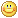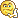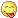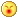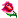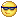نظر خصوصی
مشخصات شما ذخیره شود ؟ [حذف مشخصات] [شکلک ها]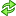کد امنیتی
اطلاعات کاربری
 نام کاربری : رمز عبور :
• فراموشی رمز عبور؟
• لینک دوستان
آرشیو
آمار سایت
• کل مطالب : 155
• کل نظرات : 0
• افراد آنلاین : 1
• تعداد اعضا : 4
• آی پی امروز : 13
• آی پی دیروز : 23
• بازدید امروز : 44
• باردید دیروز : 48
• گوگل امروز : 0
• گوگل دیروز : 0
• بازدید هفته : 92
• بازدید ماه : 1,905
• بازدید سال : 33,743
• بازدید کلی : 154,795
• کدهای اختصاصی